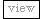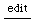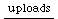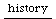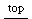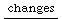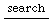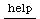## operation cannot be called from this Process.

back to error messages

### error dump

```ERROR:
Primitive '_SCView_SetProperty' failed.

operation cannot be called from this Process.
Instance of SCSlider {    (03C17BD0, gc=5C, fmt=00, flg=00, set=03)
instance variables 
dataptr : RawPointer 1B58F60
parent : instance of SCTopView (03C553E0, size=9, set=04)
action : nil
background : nil
keyDownAction : instance of Function (03C5B290, size=2, set=01)
keyUpAction : instance of Function (03C5B260, size=2, set=01)
keyTyped : nil
}
CALL STACK:
Object-verboseHalt
arg this = <instance of SCSlider>
Object-primitiveFailed
arg this = <instance of SCSlider>
SCView-setProperty
arg this = <instance of SCSlider>
arg key = 'value'
arg value = 0.200000
SCSlider-value_
arg this = <instance of SCSlider>
arg val = 0.200000
< FunctionDef in closed FunctionDef >  (no arguments or variables)
Routine-prStart
arg this = <instance of Routine>
arg inval = 2752.560649```

### how to cause it:

```//when trying to access the application clock from the
//system thread this causes this error.
//OSCresponder and Routine evaluate their functions by default in a SystemClock thread.

w = SCWindow("test", Rect(128, 64, 360, 160));
w.view.decorator = d = FlowLayout(w.view.bounds);
b = SCSlider(w, Rect(20, 50, 80, 24));
w.front;

b.value = 0.6;

Routine({ 0.1.wait; b.value = 0.2 }).play(SystemClock);```

### how to avoid it, if known

```// use the {}.defer message.

w = SCWindow("test", Rect(128, 64, 360, 160));
w.view.decorator = d = FlowLayout(w.view.bounds);
b = SCSlider(w, Rect(20, 50, 80, 24));
w.front;

b.value = 0.6;

Routine({ 0.1.wait; defer({ b.value = 0.2 }) }).play(SystemClock);```